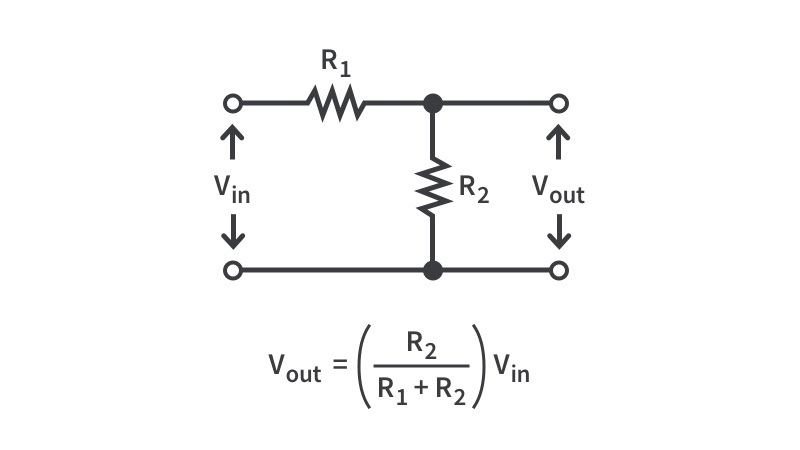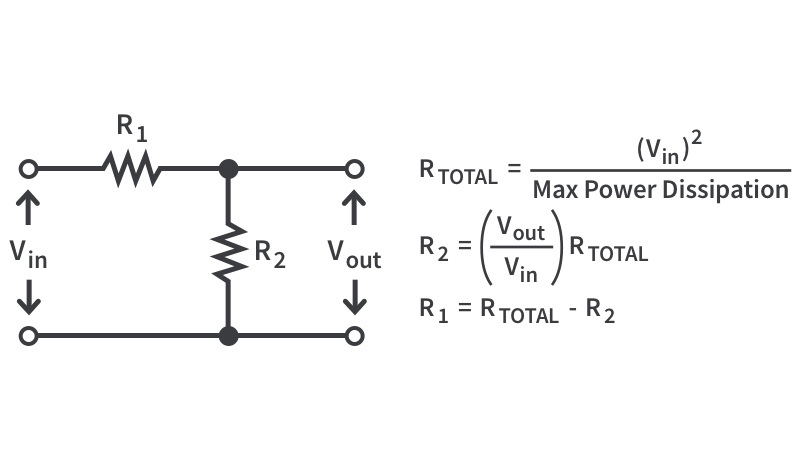# Voltage DividerInputOutputA simple, dirty way to get a lower voltage is to use a voltage divider - putting two (or more!) resistors in series and tapping the voltage between them. If you know the value of the resistors and voltage input, you can calculate the output voltage. If the resistors are the same value, then you’ll get half the input voltage. Otherwise, the math is going to be slightly more complicated but you won’t have any issues - just use the equation above and then use this fancy tool to double-check your work! Or just use the tool, we’re cool with that, too.InputOutput